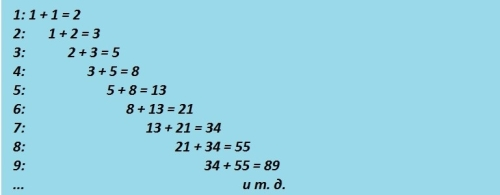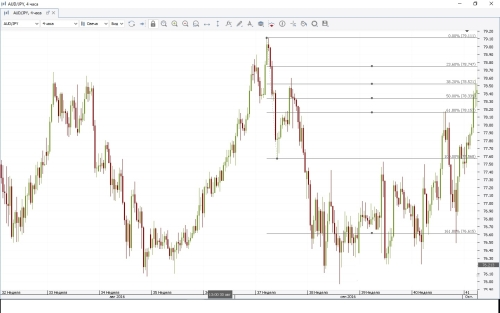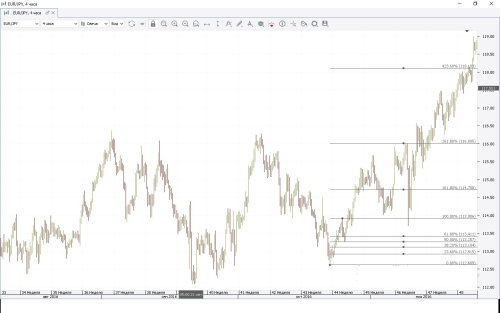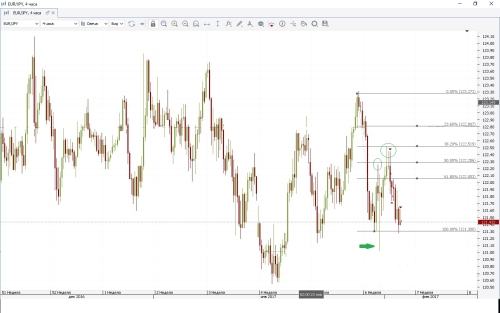Good day, my friends! In this article I will introduce you to the Fibonacci levels.
History.
Indicator Fibonacci was named after the Italian mathematician Leonardo of Pisa who lived in Europe from 1175 till 1250 year. Fibonacci was his nickname. In his "Book of the abacus," written in 1205, the scientist detail examined a series of numbers -.. 0,1,1,2, 3, 5, 8,13, 21, 34, 55, 89, 144, etc., where each subsequent number is the sum of the previous two. The so-called summatsionnuyu sequence subsequently became known as the Fibonacci numbers. Fibonacci numbers - a system of natural numbers related to each other by certain relations and built on the principle of calculation of each subsequent member of the system, starting from the third through the arithmetic addition of the two previous ones.Each of its members, beginning with the third, is the sum of the previous two, and the ratio of adjacent numbers of a number of approaches to the relationship, so-called, Golden Section, where the ratio of each of these numbers to the next member of the series tends to the value of 0.618, and the ratio of each term of the series the previous member tends to 1,618 (Fibonacci ratios).In other words, if a point divides the line into two unequal length so that the ratio of the whole length of the line to a length of greater length is the ratio of the length of the larger segment to the smaller, the dividing point - this is the "golden section". As a percentage rounded value of the golden ratio - a division of any quantity in respect of 62% and 38%. There is another law - if any member of the Fibonacci sequence is not divided on the next number, and the number by one, you get a ratio approximate to 0.382. In case if you take the third term of the series after the original, the ratio between them is approximately equal to 0.236. On the basis of these relationships have been established Fibonacci levels that can be used to analyze and predict the dynamics of the various financial instruments. Adopted schetayu that the first who decided to use the mathematical Fibonacci pattern in technical analysis of financial markets, was an American financier Ralph Nelson Elliott, famous for its wave theory. (But more on that in another article)
What is the Fibonacci levels.
Fibonacci levels allow the trader to identify possible targets of correction, as well as strong support and resistance levels. There are several variations of the use of Fibonacci method, we analyze the specific Fibonacci line. Fibonacci lines are specific graphic design in the form of a grid consisting of a trend line, and nine horizontal lines intersecting it at the points of 0.0%, 23.6%, 38.2%, 50%, 61.8%, 100%, 161 8%, 261.8% and 423.6%. The strongest levels are 38.2%, 50%, 61.8%. as they provide maximum support and resistance during the course changes. Fibonacci is a relationship between the trend and the corrective movement in 38%, 50% and 62%, thereby forming a primary levels of correction. That coincidence graphical location on the chart and Fibonacci levels can identify entry and exit levels of the market. Inflicting Fibonacci grid on schedule in the minimum and maximum point you will see how important price levels intersect with Fibonacci lines. The use of this indicator is based on the assertion that in practice, a strong rise or fall in prices, the price returns back, correcting its initial movement to some extent. When you return the price of the currency pair, as a rule, meets resistance / support in some arbitrary points. To determine the most probable location of such points and Fibonacci indicator is used that allows you to build an appropriate trading strategy. The indicator does not work in a flat market.Also widely used these lines to determine the levels of profit and the installation of stop orders. Profit is recommended to fix the Fibonacci extension levels. If the graph coincide with the level of support and resistance zones Fibonacci grid is further evidence of the significance of these levels.For an uptrend, it is necessary to do the opposite. Click on a minimum price fluctuation and drag the cursor to the maximum (see Figure).Using Fibonacci levels can be determined not only possible purpose of correction, but the possible targets in the case of continuation of trend - it is 161.8%, 261.8% and 423.6% Fibonacci levels.Trade using Fibonacci lines. Look at the picture below, we see the price rebounded from the level of 38.20%, but this price breaks the level of 61.80% and almost touching the level of 50.00% started a correction to the minimum point, but then the price returned to the level of 61.80% leaving a hollow candle (noted green arrow) and continued growth hit 50.00% level, touching the level 38.20% the price has gone down over the past two candles price tried to overcome the level of 38.20%, but as you can see on the image failed, and the price returned to the starting point. The peaks ( are marked green circle ) can we say that the price is not ready to go to the top and should expect a decrease.Trading methods: